/

### The inverse Wishart distribution

The inverse Wishart distribution (otherwise called the inverted Wishart distribution)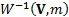is a probability distribution that is used in the Bayesian analysis of real-valued positive definite matrices (e.g. matrices of the type that arise in risk management contexts). It is a conjugate prior for the covariance matrix of a multivariate normal distribution.

It has the following characteristics, where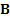is a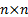matrix,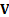is a positive definite matrix and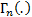is the multivariate gamma function.

 Parameters (and constraints on parameters):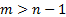(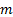= degrees of freedom, real)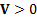(= inverse scale matrix, positive definite) Support (i.e. values that it can take)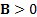, i.e. is positive definite,anmatrix Probability density function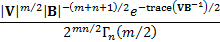Mean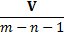If the elements ofare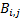and the elements ofare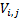then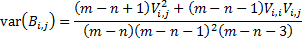The main use of the inverse Wishart distribution appears to arise in Bayesian statistics. Suppose we want to make an inference about a covariance matrix,, whose prior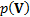has adistribution. If the observation set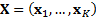where the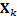are independent-variate Normal (i.e. Gaussian) random variables drawn from a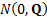distribution then the conditional distribution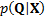, i.e. the probability of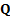given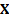, has a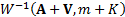distribution, where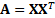is the sample covariance matrix.

The univariate special case of the inverse Wishart distribution is the inverse gamma distribution. With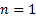,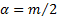,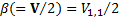,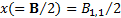we have: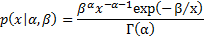where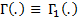is the ordinary (i.e. univariate) Gamma function, see MnGamma.

For other probability distributions see here.